Q:

# Count of Subarrays

Given an array of N positive integers a1, a2,  ..., an. The value of each contiguous subarray of a given array is the maximum element present in that subarray. The task is to return the number of subarrays having value strictly greater than K.

Input:

The first line contains two space-separated positive integers N and K denoting the size of the array and the value of K. The second line contains N space-separated positive integers denoting the elements of the array.

Output:

Output the number of subarrays having value strictly greater than K.

Constraints:

```1 <= T <= 50
1 <= N <= 100
1 <= a[i] <= 105
```

Example:

```Test case: 1

Input:
5 3
3 4 5 2 7

Output:
13

Test case: 2
4 1
1 2 3 4

Output:
9
```

Explanation:

```Test case 1:
All possible subarrays are listed below
with their respective value (maximum in the subarray)

3 -> 3
4 -> 4
5 -> 5
2 -> 2
7 -> 7
3, 4 -> 4
4, 5 -> 5
5, 2 -> 5
2, 7 -> 7
3, 4, 5 -> 5
4, 5, 2 -> 5
5, 2, 7 -> 7
3, 4, 5, 2 -> 5
4, 5, 2, 7 -> 7
3, 4, 5, 2, 7 -> 7

So, number of valid subarrays is 13```

1. Create dp[n][n] to store value (maximum) of subarray;
2. Initialize count = 0 which will be our final result;
3. Base case computation (single length subarrays),
```for i=0 to n
// since only one element in single length subarray,
// just check for that element
if(a[i]>k)
dp[i][i]=a[i];
count++;
else
dp[i][i]=-1; // or arr[i] itslef
end for
```
4. Computing all length subarray cases,
```for subarray length,len=2 to n
for start=0 to n-len
end=start+len-1; // last element of subarray
if(a[end]>k || dp[start][end-1]>k)
dp[start][end]=std::max(a[end],dp[start][end-1]);
count++;
else
dp[start][end]=-1;
end for
end for
```

Okay, so the strategy is to compute for subarray a[start..end] with help of already computed one a[start..end-1].

Subarray a[start..end] will only make a count if a[start..end-1] already makes a count ( yes because, it's part of the subarray so, anything max in it would be max in the parent one too) Or if a[end]>k.

In both the cases maximum of the subarray, a[start..end] is greater than K

That's what we have done.

Below is illustration for our test case 1,

Input array: [3, 4, 5, 2, 7] and K=3.

So, let's compute the basic test case first

We need a 5X5 DP array for this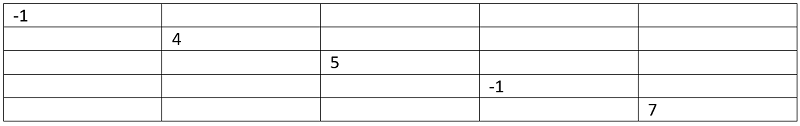Starting to compute for other values.

Len=2

Start=0, end=1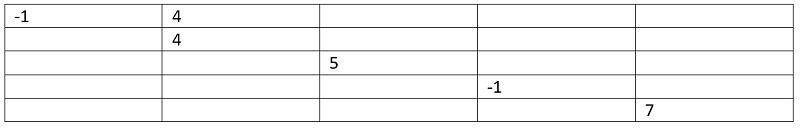Start=1, end=2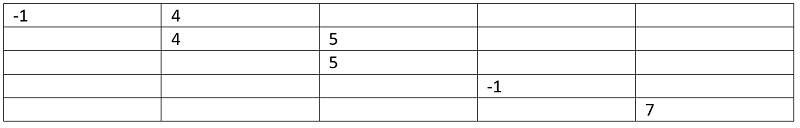Start=2, end=3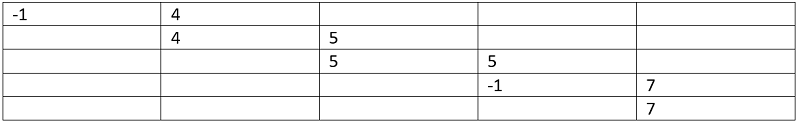Start=3, end=4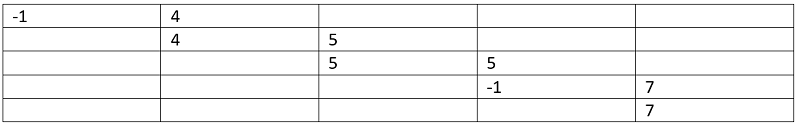Len=3

Start=0, end=2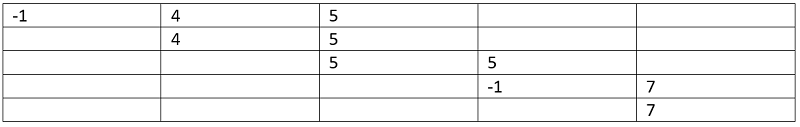Start=1, end=3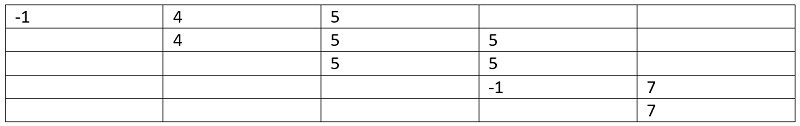Start=2, end=4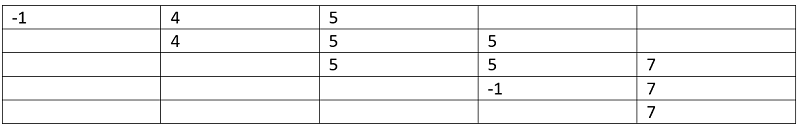Len=4

Start=0, end=3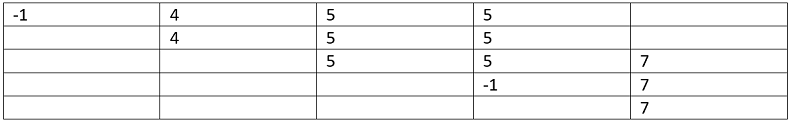Start=1, end=4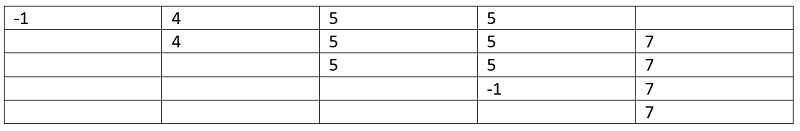Len=5

Start=0, end=3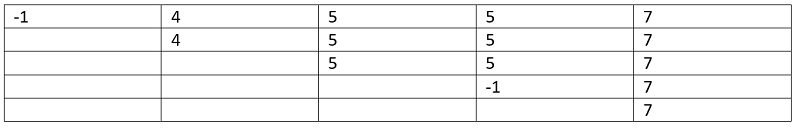Done!

Count is 13 (count of positive values in the array).

C++ Implementation:

``````#include <bits/stdc++.h>
using namespace std;

int maximum(int a, int b)
{
return (a > b) ? a : b;
}

int subArray(vector<int> a, int n, int k)
{

int dp[n][n];
int count = 0;
for (int i = 0; i < n; i++) {
if (a[i] > k) {
dp[i][i] = a[i];
count++;
}
else
dp[i][i] = -1; //or arr[i] itslef
}

for (int len = 2; len <= n; len++) {
for (int start = 0; start <= n - len; start++) {
int end = start + len - 1;
if (a[end] > k || dp[start][end - 1] > k) {
dp[start][end] = std::max(a[end], dp[start][end - 1]);
count++;
}
else
dp[start][end] = -1;
}
}

return count;
}

int main()
{
int n, item, k;

cout << "Input size of array\n";
cin >> n;

cout << "Input k\n";
cin >> k;

cout << "Add the array elements\n";
vector<int> a;

for (int j = 0; j < n; j++) {
scanf("%d", &item);
a.push_back(item);
}

cout << "Total count of valid subarray is " << subArray(a, n, k) << endl;

return 0;
}``````

Output:

```Input size of array
5
Input k
3
3 4 5 2 7
Total count of valid subarray is 13```